# Common Core: 6th Grade Math : Describe the Nature of the Attribute Under Investigation: CCSS.Math.Content.6.SP.B.5b

## Example Questions

← Previous 1

### Example Question #1 : Describe The Nature Of The Attribute Under Investigation: Ccss.Math.Content.6.Sp.B.5b

Several students from Jones Middle School participated in a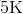race this weekend. The gym teacher at the school displayed the time, in minutes, it took each student to complete the race in the chart provided. What is the attribute being measured in this chart?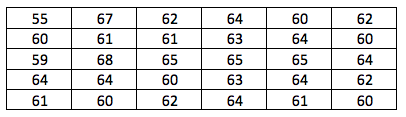The distance that a runner in arace ran

The heart rate of runners during arace

The length of time it took to start arace

The length of time it took to complete arace

The length of time it took to complete arace

Explanation:

To answer this question, we need to make sure we've read the text provided in the question:

"Several students from Jones Middle School participated in arace this weekend. The gym teacher at the school displayed the time, in minutes, it took each student to complete the race in the chart provided. "

Based on the information given, the length of time it took to complete arace is being measure in the table.

### Example Question #2 : Describe The Nature Of The Attribute Under Investigation: Ccss.Math.Content.6.Sp.B.5b

Several students from Jones Middle School participated in arace this weekend. The gym teacher at the school displayed the time, in minutes, it took each student to complete the race in the chart provided. What is the the unit of measurement in this chart?Hours

Minutes

Seconds

No unit of measurement is given in the chart

Minutes

Explanation:

To answer this question, we need to make sure we've read the text provided in the question:

"Several students from Jones Middle School participated in arace this weekend. The gym teacher at the school displayed the time, in minutes, it took each student to complete the race in the chart provided. "

Based on the information given, the unit of measurement used in this chart is minutes.

### Example Question #3 : Describe The Nature Of The Attribute Under Investigation: Ccss.Math.Content.6.Sp.B.5b

Several students from Jones Middle School participated in arace this weekend. The gym teacher at the school displayed the time, in minutes, it took each student to complete the race in the chart provided. How many observations were made?Explanation:

The number of observations made represents the number of students that participated in the race.The chart shows how long it took each student to complete arace. Each entry in the chart is how long it took each student to complete the race, so we can count up the number of entries to answer this question.

If done correctly, you should have countedentries; thus,students participated in the race.

### Example Question #4 : Describe The Nature Of The Attribute Under Investigation: Ccss.Math.Content.6.Sp.B.5b

Wrigley, a chocolate labradoodle, had a litter of puppies. During a veterinary appointment, each of the puppies were weighed, in pounds, and each weight was documented on the chart provided. What is the attribute being measured in this chart?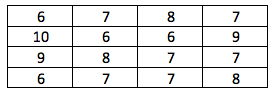The weight of each puppy

The height of each puppy

The number of puppies in each litter

The age of each puppy

The weight of each puppy

Explanation:

To answer this question, we need to make sure we've read the text provided in the question:

"Wrigley, a chocolate labradoodle, had a litter of puppies. During a veterinary appointment, each of the puppies were weighed, in pounds, and each weight was documented on the chart provided. "

Based on the information given, the weight of each puppy is being measure in the table.

### Example Question #5 : Describe The Nature Of The Attribute Under Investigation: Ccss.Math.Content.6.Sp.B.5b

Wrigley, a chocolate labradoodle, had a litter of puppies. During a veterinary appointment, each of the puppies were weighed, in pounds, and each weight was documented on the chart provided. What is the the unit of measurement in this chart?Weight

Inches

Pounds

Ounces

Pounds

Explanation:

To answer this question, we need to make sure we've read the text provided in the question:

"Wrigley, a chocolate labradoodle, had a litter of puppies. During a veterinary appointment, each of the puppies were weighed, in pounds, and each weight was documented on the chart provided. "

Based on the information given, the unit of measurement used in this chart is pounds.

### Example Question #6 : Describe The Nature Of The Attribute Under Investigation: Ccss.Math.Content.6.Sp.B.5b

Wrigley, a chocolate labradoodle, had a litter of puppies. During a veterinary appointment, each of the puppies were weighed, in pounds, and each weight was documented on the chart provided. How many observations were made?Explanation:

The number of observations made represents the number of puppies in the litter whose weight was recorded.The chart shows the weight of each puppy, so we can count the entires to find the correct answer.

If done correctly, you should have countedentries; thus,puppies's weights were recorded.

### Example Question #7 : Describe The Nature Of The Attribute Under Investigation: Ccss.Math.Content.6.Sp.B.5b

A teacher decided to start tracking the amount of time her students spent on homework each week. The data is displayed in the histogram provided. What is the attribute being measured in this chart?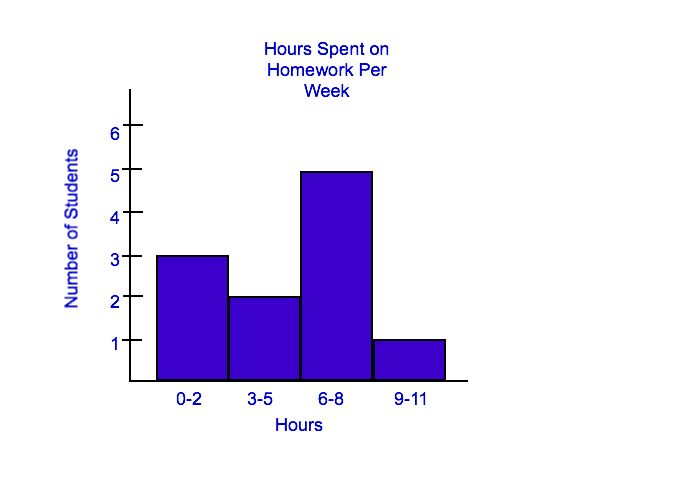Teacher's Chart

Number of Students

Hours Spent on Homework Per Week

Hours

Hours Spent on Homework Per Week

Explanation:

When we are given a graph or a chart that is labeled with a title, that is generally the best place to start when looking for what is being measured in a chart. This graph is labeled with "Hours Spent on Homework Per Week". Also, when we read the text before the question, we were told that, "a teacher decided to start tracking the amount of time her students spent on homework each week. The data is displayed in the histogram provided"; thus, hours spent on homework per week is the best answer choice.

### Example Question #1 : Describe The Nature Of The Attribute Under Investigation: Ccss.Math.Content.6.Sp.B.5b

A teacher decided to start tracking the amount of time her students spent on homework each week. The data is displayed in the histogram provided. What is the the unit of measurement in this chart?Weeks

Studnets

Hours

Minutes

Hours

Explanation:

To answer this question correctly, we need to look at the histogram. The teacher is tracking hours that each student spent on homework per week; thus, the correct answer is hours.

### Example Question #9 : Describe The Nature Of The Attribute Under Investigation: Ccss.Math.Content.6.Sp.B.5b

A teacher decided to start tracking the amount of time her students spent on homework each week. The data is displayed in the histogram provided. How many observations were made?Explanation:

For this plot, the number of observations made represents the number of students that participated in the survey. Each bar goes up to a certain number of students, so we can add up the height of each bar to determine the number of observations that were made.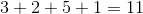If done correctly,  we should have found thatstudents were surveyed.

### Example Question #10 : Describe The Nature Of The Attribute Under Investigation: Ccss.Math.Content.6.Sp.B.5b

Mrs. Hobb's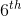grade class just completed a fundraiser to help fund their field trips for this school year. The money that the students sold is displayed in the histogram provided. What is the attribute being measured in this chart?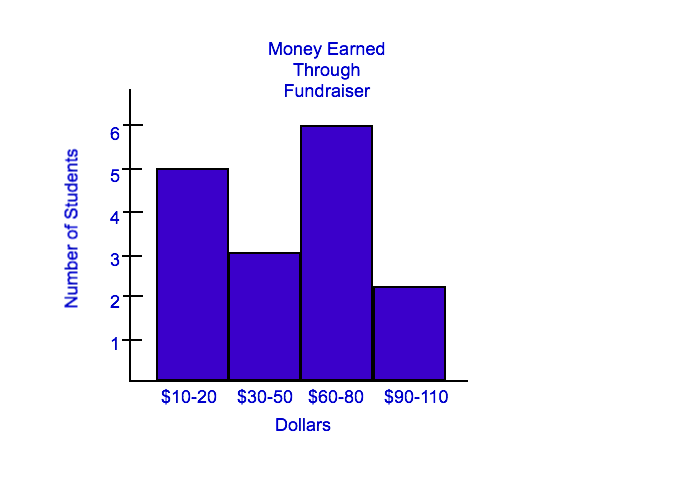Number of Students who Sold for the Fundraiser

Dollars

Number of Students

Money Earned Through the Fundraiser

When we are given a graph or a chart that is labeled with a title, that is generally the best place to start when looking for what is being measured in a chart. This graph is labeled with "Money Earned Through Fundraiser". Also, when we read the text before the question, we were told that, "Mrs. Hobb'sgrade class just completed a fundraiser to help fund their field trips for this school year. The money that the students sold is displayed in the histogram provided"; thus, money earned through the fundraiser is the best answer choice.